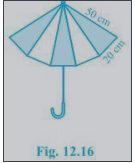# NCERT Solutions Class 9 Mathematics Solutions for Heron’s Formula - Exercise 12.2 in Chapter 12 - Heron’s Formula

An umbrella is made by stitching 10 triangular pieces of cloth of two different colors (see

Fig.12.16), each piece measuring 20 cm, 50 cm, and 50 cm. How much cloth of each color is

required for the umbrella?For each triangular piece, The semi perimeter will be

s = (50 + 50 + 20)/2 cm = 120/2 cm = 60cm

Using Heron's formula,

Area of the triangular piece = √[s (s-a) (s-b) (s-c)]

\begin{aligned} &=v[60(60-50)(60-50)(60-20)] \mathrm{cm}^{2}\\ &=\sqrt{[60 \times 10 \times 10 \times 40] \mathrm{cm}^{2}}\\ &=200 \mathrm{v} 6 \mathrm{cm}^{2}\\ &\therefore \text { The area of all the triangular pieces }=5 \times 200 \sqrt{6} \mathrm{cm}^{2}=1000 \mathrm{v} 6 \mathrm{cm}^{2} \end{aligned}

Video transcript
"hello students welcome to leader q a video session i am seth your math tutor and question for today is an umbrella is made by stitching 10 triangular pieces of cloth of two different colors see the figure 12.16 each piece measuring 20 centimeter 50 centimeter and 50 centimeter remember there are 10 triangular pieces of each of this how much cloth of each color is required for the umbrella so we need to find how much area is required so here first of all let us consider the semi parameter semi perimeter of each triangle that is s will be equal to 50 plus 50 plus 20 divided by 2 hence semi perimeter is 60 centimeter now you can find the area for each of this triangle piece by using harrow's formula so using heron's formula area of the triangle piece that will be equal to root of s s minus a s minus b and s minus c so that will be equal to root of that is 60 60 minus 50 again 60 minus 50 and 60 minus 20. and calculating it will give you root of 16 into 10 into 10 into 40. so your final answer should be 200 root 6 centimeter square and that is your area we will indicate it with letter a so here i'll continue that area comes out to be that is a 200 root 6 centimeter square so area of all triangle pieces can be multiplied by multiplying the total available pieces in the umbrella so so as you can see there are five triangle pieces so 5 into area of each piece that is 200 root 6 and that is 5 into 200 root 6 that comes out to be thousand root 6 and centimeter square so that is our final answer if you have any query you can drop it in our comment section and subscribe to lido for more such query thank you for watching "
Related Questions

Lido

Courses

Teachers

Book a Demo with us

Syllabus

Maths
CBSE
Maths
ICSE
Science
CBSE

Science
ICSE
English
CBSE
English
ICSE
Coding

Terms & Policies

Selina Question Bank

Maths
Physics
Biology

Allied Question Bank

Chemistry
Connect with us on social media!